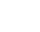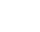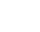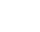Language:
• 86-15253927511
Home / All / FAQ /

FAQs

# Fluid power formulas

Update Time:2022/12/15

Bore Diameter = Inside Tube Circumference / 3.1415

Rod Diameter = Rod Circumference / 3.1415

Stroke = Extended Length (center to center of pins) – Retracted Length (center to center of pins)

Volume Displacement in gallons = (Bore Radius² * 3.1415) * Stroke / 231

Fluid Pressure (PSI) = Force (lbs) / (Bore Radius² * 3.1415)

Force (lbs) = (Bore Radius² * 3.1415) * Pressure (PSI)

Fluid Flow Rate (GPM) = Volume displacement (gallons) / Time units (minutes)

Hydraulic Horsepower = pressure (PSI) * Fluid Flow Rate (GPM) / 1714

Cylinder Extending Speed(Inch per second) = (231 * GPM) / (60 * Bore Radius² * 3.1415)

Do you want to quickly know the product quotation you need?
•Established in 2008
•24/7 Service
•12 Months Waranty
•Customize Available
subscription
KEEP IN TOUCH